6.041SC | Fall 2013 | Undergraduate

# Probabilistic Systems Analysis and Applied Probability

## Unit I: Probability Models And Discrete Random Variables

« Previous | Next »

This unit covers the basic framework of probability theory: probabilistic models, conditional probabilities, independence, the Bayes’ rule, and counting methods. In addition, it introduces discrete random variables and the concept of the Probability Mass Function (PMF) used to describe the probability distribution of one or several random variables. Finally, it defines the concepts of expectation and variance, and their basic properties.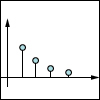Lecture 1: Probability Models and Axioms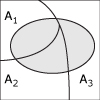Lecture 2: Conditioning and Bayes’ Rule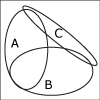Lecture 3: IndependenceLecture 4: Counting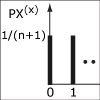Lecture 5: Discrete Random Variables; Probability Mass Functions; Expectations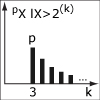Lecture 6: Discrete Random Variable Examples; Joint PMFs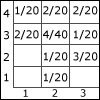Lecture 7: Multiple Discrete Random Variables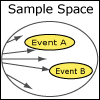Quiz 1

Looking for something specific in this course? The Resource Index compiles links to most course resources in a single page.

« Previous | Next »

## Course Info

Fall 2013
##### Learning Resource Types
Lecture Videos
Recitation Videos
Problem Sets with Solutions
Exams with Solutions